# I need all parts answered please! If you can'tdon't answera.Express the following waveforms as functions of time in piece-wise fashion.w(t)r(t)2-6-3-4b.Express the above waveforms in terms of shifted and weighted sums of r(t) = tu(t) and u(t).C.Convolve the two waveforms.above.

Question
1 views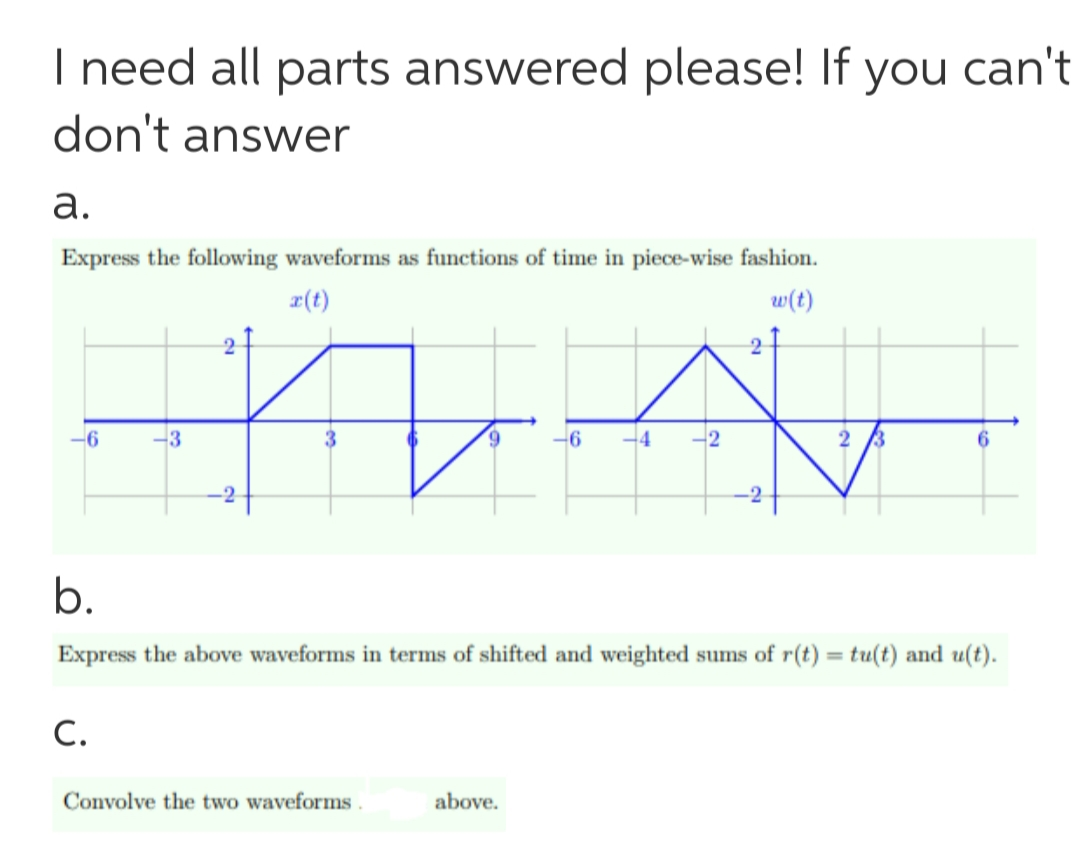help_outlineImage TranscriptioncloseI need all parts answered please! If you can't don't answer a. Express the following waveforms as functions of time in piece-wise fashion. w(t) r(t) 2 -6 -3 -4 b. Express the above waveforms in terms of shifted and weighted sums of r(t) = tu(t) and u(t). C. Convolve the two waveforms. above. fullscreen
check_circle

(a) Piece-wise Fashion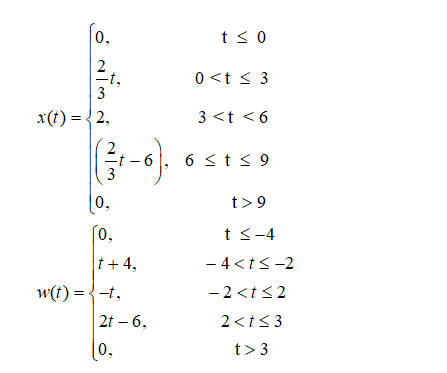(b) Shifted and weighted terms: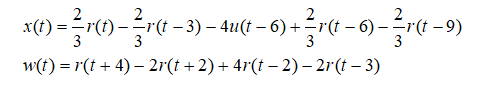(c) Convolution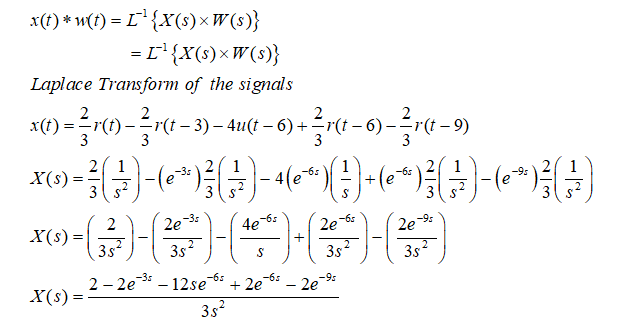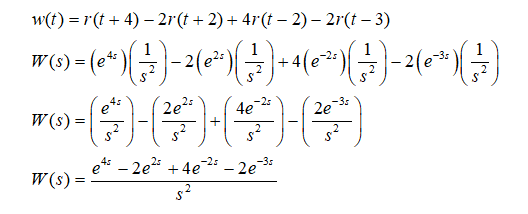...

### Want to see the full answer?

See Solution

#### Want to see this answer and more?

Solutions are written by subject experts who are available 24/7. Questions are typically answered within 1 hour.*

See Solution
*Response times may vary by subject and question.
Tagged in